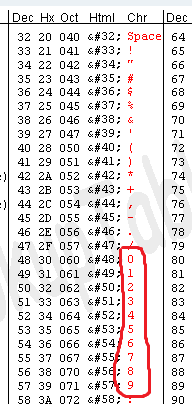C language question/ printing functions

8 posts / 0 new
Author
Message

Hello guys,

There is a bit of a question mark inside of me related to this chunk of code. In function transmitByte(.................) what >>>'0'<<< this is doing and why its applied here.

void printFloat(float number) {
number = round(number * 100) / 100;                               /* round off to 2 decimal places */
transmitByte('0' + number / 10);                                 /* tens place */
transmitByte('0' + number - 10 * floor(number / 10));           /* ones */
transmitByte('.');
transmitByte('0' + (number * 10) - floor(number) * 10);        /* tenths */
/* hundredths place */
transmitByte('0' + (number * 100) - floor(number * 10) * 10);
printString("\r\n");
}

maybe its of a more trivial question  but my search results did not make it clear to me.

work in progress...

You add '0' to convert binary values (0..9) to displayable characters '0'..'9'.

This is because, in ASCII, the things that display as '0' to '9' do not have character numbers 0..9 but as you see here:

http://www.asciitable.com/They have character numbers 48 to 57 (aka 0x30 .. 0x39). So to make 0..9 display as '0' to '9' you either add 48 or 0x30 or '0' (which are all exactly the same thing).

BTW is there some reason not to use %f print formats in (s)printf() ?

now it makes sense to me why the transmitByte() was behaving erratically in Terminal,  when I tried to print out numerical values because I was doing it without the   '  '  those upper brackets. So every time printing numerical values I should use the 'value' construction.

Actually I decided to try this way to learn a workaround in case AVR floating point libraries would not be available.

work in progress...

I don't understand your question but the EASIEST way to print things is to use printf(). Anything else just looks like unnecessary over-complication.

for example in this expression:

transmitByte('0' + number / 10);

( '0' + number/10) = lets consider number/10 is 7, so if we rewrite it ('0' + 7) = how C is evaluating this?, are they added like normal numbers? 0+7?

and what if the "number " has fractions? does the same rules apply?

work in progress...

Dave_Zeb. wrote:

for example in this expression:

transmitByte('0' + number / 10);

( '0' + number/10) = lets consider number/10 is 7, so if we rewrite it ('0' + 7) = how C is evaluating this?, are they added like normal numbers? 0+7?

and what if the "number " has fractions? does the same rules apply?

In this example the compiler adds the ASCII CHARACTER zero (aka ASCII code 0x30 which is "the number zero on your keyboard") to the NUMBER 7 which in turn equals the NUMBER 0x37 which is also the ASCII CHARACTER seven (aka ASCII code 0x37 which is "the number seven on your keyboard").

Adding the character '0' to a decimal number from 0..9 is a trick to convert a decimal number from 0..9 to the ASCII character '0'..'9'.  All three of these functions do the same thing:

char DecimalDigitToASCIICharacter(int Digit)
{
char Retval;

if (Digit >= 0 && Digit <= 9) {
Retval = '0' + Digit;           // converts decimal digit 0..9 to ASCII character '0'..'9'
} else
Retval = -1;
}

return Retval;
}

char DecimalDigitToASCIICharacter2(int Digit)
{
char Retval;

switch (Digit) {
case 0:
Retval = '0';               // converts decimal 0 to ASCII character '0' (aka 0x30)
break;
case 1:
Retval = '1';
break;
case 2:
Retval = '2';
break;
case 3:
Retval = '3';
break;
case 4:
Retval = '4';
break;
case 5:
Retval = '5';
break;
case 6:
Retval = '6';
break;
case 7:
Retval = '7';               // converts decimal 7 to ASCII character '7' (aka 0x37)
break;
case 8:
Retval = '8';
break;
case 9:
Retval = '9';
break;
default:
Retval = -1;    // invalid input
break;
}

return Retval;
}

char DecimalDigitToASCIICharacter3(int Digit)
{
return '0' + (Digit % 10);          // converts decimal digit 0..9 to ASCII character '0'..'9', mod operation limits input to 0..9
}

Last Edited: Mon. Nov 12, 2018 - 03:42 PM

and what if the "number " has fractions?

You need to get hold on a C book!

in this case what / and % means for integers

Your entire thing here could be:

void printFloat(float number) {
char buff;
sprintf(buff, "%05.2f\r\n", number);
printString(buff);
}

Why over complicate things? In fact it could be even easier as:

void printFloat(float number) {
printf("%05.2f\r\n", number);
}

if you previously had used FDEV_SETUP_STREAM to connect transmitByte() to the stdout output stream.

Here's a short example to show what %05.2f delivers:

#include <stdio.h>
#include <stdlib.h>

int main(void) {
float a,b,c;
for (int i = 0; i < 10; i++) {
b = rand();
c = rand();
if (c != 0) {
a = b / c;
}
else {
a = 3.14159;
}
printf("c = %05.2f\n", a);
}
}

outputs:

D:\c>printf.exe
c = 00.00
c = 00.24
c = 01.22
c = 00.39
c = 01.10
c = 00.20
c = 01.38
c = 20.29
c = 00.25
c = 00.89